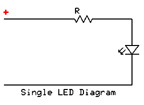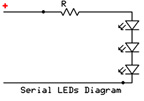# LED Resistor Calculator

Voltage drop is usually 1.9~2.1V for AlGaInP, 3.1~3.5 for InGaN and 1.2V for Infrared..

Current is usualy 20mA, for UFO LEDs current is: 30mA for InGaN and 50mA for AlGaInP.

Supply Voltage - depends what you have at disposal. Usuall is 6V or 12V source.
 Single led:Supply Voltage VOLTS Voltage Drop Across LED VOLTS Desired LED Current MILLIAMPS Calculated Limiting Resistor OHMS Nearest higher rated 10% resistor Calculated Resistor Wattage WATTS Safe pick is a resistor with power rating of WATTS Leds in series:Supply Voltage VOLTS Voltage Drop Across LED VOLTS Desired LED Current MILLIAMPS How many leds connected Calculated Limiting Resistor OHMS Nearest higher rated 10% resistor Calculated Resistor Wattage WATTS Safe pick is a resistor with power rating of WATTS Leds in parallel:Supply VoltageSupply Voltage VOLTS Voltage Drop Across LED VOLTS Desired LED Current MILLIAMPS How many leds connected Calculated Limiting Resistor OHMS Nearest higher rated 10% resistor Calculated Resistor Wattage WATTS Safe pick is a resistor with power rating of WATTS